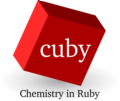back to Modules

# cuby_shell

This executable is an extended version of ruby's irb interactive shell. It starts with preloaded cuby framework.

The interactive shell is useful for many simple tasks, such as:

### Calculator

The shell can serve as a powerful calculator with ruby syntax. Cuby's classes Matrix and Vector can be used for linear algebra. Example:

``````irb(main):001:0> r = 15.0
=> 15.0
irb(main):002:0> 2.0 * Math::PI * r**2
=> 1413.71669411541
irb(main):003:0> m = Matrix[[1,2],[3,4]]
=>
|  1.00  2.00 |
|  3.00  4.00 |
irb(main):004:0> v = Vector[1,2]
=>
Vector: [1.0, 2.0]
irb(main):005:0> (m * v).to_vector
=>
Vector: [5.0, 11.0]
irb(main):006:0> (m.transpose - m.inverse) * 10
=>
| 30.00 20.00 |
|  5.00 45.00 |``````

### Unit conversion

Cuby contains constants for conversion of units used in computational chemistry and selected physical constants. See file classes/constants.rb for reference.

``````irb(main):001:0> 1.5 * KCAL2HARTREE
=> 0.00239040205765809
irb(main):002:0> 15 * ANGSTROM2BOHR
=> 28.3458920088188
irb(main):003:0> SPEED_OF_LIGHT_SI
=> 299792458.0``````

### Running calculations

``````irb(main):001:0> job = Job.create('input.yaml', 'water.xyz')
=> ...
irb(main):002:0> job.run
Running prepare...
Energy: -2558.54567040571 kcal/mol
=> -2558.54567040571``````